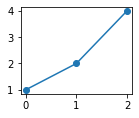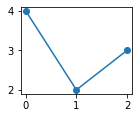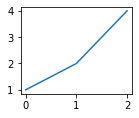# 2 Image

In this section, we study how to embed images created by Matplotlib, Bokeh, and Altair libraries.

## 2.1 Matplotlib

First, `figsize` of `figure` is set to `[2, 1.6]` to show small figures for saving space.

``````import matplotlib
import matplotlib.pyplot as plt
matplotlib.rcParams['figure.figsize'] = [2, 1.6]``````

 2020-12-20 08:15:13 (12.1ms) python3 (901ms)

A call of `plt.plot` generates a standard plain text output (a list of `Line` object in this example) and a PNG image as display data:

``plt.plot([1, 2, 4], marker='o')``

 2020-12-20 08:15:13 (62.0ms) python3 (963ms)

``[<matplotlib.lines.Line2D at 0x2119a5d3160>]``If you want to hide the input source and the plain text output, you can use a `inline` option to show just the display data such as an image or HTML:

`````````python inline
plt.plot([4, 2, 3], marker='o')
`````````"Inline code" is useful to display a plot in shorthand notation. `{{plt.plot([1, 2, 4])}}` generates:Note that the plain text output (`[<matplotlib.lines...]`) does not displayed if the inline code generates display data.

## 2.2 Bokeh

You can embed Bokeh's plots into MkDocs HTML documents. Following User Guide "Embedding Plots and Apps" from the official Bokeh documents, Pheasant automatically adds extra stylesheet and javascript in HTML source. You don't need to configure `extra_css` and `extra_javascript` in your `mkdocs.yml` manually.

In order to embed plots in your document, `bokeh.embed.components` function can be used. This function returns `<script>` and `<div>` tags in HTML format. For example:

``````from bokeh.plotting import figure
from bokeh.embed import components

plot = figure(plot_width=250, plot_height=250)
plot.circle([1, 2, 3, 4, 5], [1, 3, 3, 2, 4], size=10)
script, div = components(plot)
print('[script]:', script[:140].strip(), '...')
print('[div]:', div[:40].strip(), '...')``````

 2020-12-20 08:15:13 (25.8ms) python3 (1.11s)

``````[script]: <script type="text/javascript">
(function() {
var fn = function() {
Bokeh.safely(function() {
(func ...

Pheasant uses this functionality inside automatically. You can get a Bokeh plot just write `{{plot}}`:

## 2.3 Altair

From official Example Gallery

### Simple Bar Chart

``````import altair as alt
import pandas as pd

source = pd.DataFrame({
'a': ['A', 'B', 'C', 'D', 'E', 'F', 'G', 'H', 'I'],
'b': [28, 55, 43, 91, 81, 53, 19, 87, 52]
})

alt.Chart(source).mark_bar().encode(x='a', y='b')``````

 2020-12-20 08:15:13 (28.5ms) python3 (1.15s)

### Multi-Line Tooltip

``````import altair as alt
import pandas as pd
import numpy as np

np.random.seed(42)
source = pd.DataFrame(np.cumsum(np.random.randn(100, 3), 0).round(2),
columns=['A', 'B', 'C'], index=pd.RangeIndex(100, name='x'))
source = source.reset_index().melt('x', var_name='category', value_name='y')

# Create a selection that chooses the nearest point & selects based on x-value
nearest = alt.selection(type='single', nearest=True, on='mouseover',
fields=['x'], empty='none')

# The basic line
line = alt.Chart().mark_line(interpolate='basis').encode(
x='x:Q',
y='y:Q',
color='category:N'
)

# Transparent selectors across the chart. This is what tells us
# the x-value of the cursor
selectors = alt.Chart().mark_point().encode(
x='x:Q',
opacity=alt.value(0),
nearest
)

# Draw points on the line, and highlight based on selection
points = line.mark_point().encode(
opacity=alt.condition(nearest, alt.value(1), alt.value(0))
)

# Draw text labels near the points, and highlight based on selection
text = line.mark_text(align='left', dx=5, dy=-5).encode(
text=alt.condition(nearest, 'y:Q', alt.value(' '))
)

# Draw a rule at the location of the selection
rules = alt.Chart().mark_rule(color='gray').encode(
x='x:Q',
).transform_filter(
nearest
)

# Put the five layers into a chart and bind the data
alt.layer(line, selectors, points, rules, text,
data=source, width=400, height=300)``````

 2020-12-20 08:15:13 (61.0ms) python3 (1.21s)